# Rotational forces Civil Engineering (CE) Notes | EduRev

## Civil Engineering (CE) : Rotational forces Civil Engineering (CE) Notes | EduRev

The document Rotational forces Civil Engineering (CE) Notes | EduRev is a part of the Civil Engineering (CE) Course Introduction to Dynamics and Vibrations- Notes, Videos, MCQs.
All you need of Civil Engineering (CE) at this link: Civil Engineering (CE)

6.5 Rotational forces – review of moments exerted by forces and torques

You can find a detailed discussion of forces and moments, with lots of examples, in Section 2 of these notes.   Moments and torques don’t come up very often in particle dynamics, but play a very important role in rigid body dynamics. We therefore review the most important concepts related to torques and moments here.

You need to remember, and understand, these ideas:

1. A moment is a generalized force that causes an object to rotate (see section 2). (2) A force can exert a moment on a rigid body.   The moment of a force (about the origin) is defined as M = r ×F
2. In general, a force causes a rigid body to accelerate, and will also induce an angular acceleration (so it influences both translational and rotational motion).
3. A ‘torque’ or ‘pure moment’ is a special kind of generalized force that causes an object to rotate, but has no effect on its translational motion.  As an example, a motor shaft (eg the bit on a powerdriven screwdriver!) will exert a torque on the object connected to it.
4. A torque or pure moment is a vector quantity – it has magnitude and direction.  The direction indicates the axis associated with its rotational force (following the right hand screw convention); the magnitude represents the intensity of the rotational force. The magnitude of a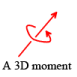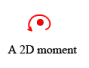6.5.1 Rate of work done by a torque or moment: If a torque Q = Qx i +Qy j + Qzk acts on an object that rotates with angular velocity ω , the rate of work done on the object by Q is

P = Q⋅ω = Qxωx +Qyωy +Qzωz

6.5.2 Torsional springs

A solid rod is a good example of a torsional spring. You could take hold of the ends of the rod and twist them, causing one end to rotate relative to the other.   To do this, you would apply a moment or a couple to each end of the rod, with direction parallel to the axis of the rod.   The angle of twist increases with the moment.  Various torsion spring designs used in practice are shown in the picture – the image is from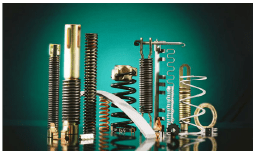More generally, a torsional spring resists rotation, by exerting equal and opposite moments on objects connected to its ends.  For a linear spring the moment is proportional to the angle of rotation applied to the spring.

The figure shows a formal free body diagram for two objects connected by a torsional spring.  If object A is held fixed, and object B is rotated through an angle θ about an axis parallel to a unit vector n, then the spring exerts a moment

Q = −κθn

on object B where κ is the torsional stiffness of the spring.   Torsional stiffness has units of Nm/radian.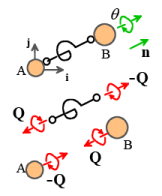The potential energy of the moments exerted by the spring can be determined by computing the work done to twist the spring through an angle θ .

1. The work done by a moment Q due to twisting through a very small angle dθ about an axis parallel to a vector n is dW = Q ⋅ dθ n
2. The potential energy is the negative of the total work done by M, i.e.
•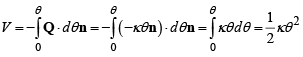A potential energy cannot usually be defined for most concentrated moments, because rotational motion is itself path dependent (the orientation of an object that is given two successive rotations depends on the order in which the rotations are applied).

Offer running on EduRev: Apply code STAYHOME200 to get INR 200 off on our premium plan EduRev Infinity!

## Introduction to Dynamics and Vibrations- Notes, Videos, MCQs

20 videos|53 docs

,

,

,

,

,

,

,

,

,

,

,

,

,

,

,

,

,

,

,

,

,

;